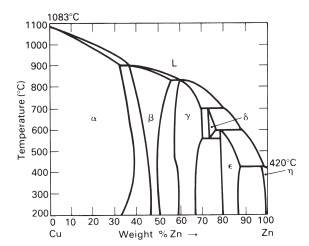# I don't understand the concept of phase diagrams?

PhyIsOhSoHardLet's use the material brass as an example.

My problem is that I have no understanding of the concept of "phases". In the phase diagram for brass, we have α, β, γ, δ, ε, and η phases as well as the liquid phase (L).

My question is, how do I interpret these phases? What is the difference between the phases?
If I have a brass material that consists of the α + β phase, what does that then tell me? And how is that different from the γ + δ phase?